# not sure I fully understand common mode and differential mode voltages

#### MikeJacobs

Joined Dec 7, 2019
162
So this a concept I have been struggling with for a long time

I found what I thought was a decent video but the issues is, every time someone tries to talk about common mode they go into some deep dark amplifier discussion. Whiping out any hope of learning what common mode is.
Here is a video I found I thought was ok

However now im confused again. Does anyone have any better resources to explain what the heck common mode voltage is.

Here is the main reason I ask. When trying to spec a part I see people always ask the question. What is the common mode range.

I don't how that question pertains to the video link I posted.
For example, I was trying to understand what is the lowest value a comparator can detect reliably.
And someone asked me, well what is the common mode voltage of the part?
So I went and looked and gave an answer they said yep you can detect down to that point.

So how do these two things connect. How does this video about common mode voltage apply to parts and this example I gave on the comparator spec?

apparently I am significantly confused here or at least feel like I am

Joined Mar 10, 2018
4,057
"Normally" when discussing common mode (CM) it implies talking about a circuit
with 2 or more nodes that "stuff" is connected to.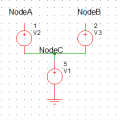So in this circuit NodeA and NodeB are some nodes in a circuit which has components
or connections attached to some other components or connections.

VnodeA = V1 + V2
VnodeB = V1 + V3

As you can see V1, its potential, is common to both NodeA and NodeB.

Sometimes we are interested in just measuring V2 or V3 or V2 - V3 or V3 - V2.
Say V2 and V3 are T sensors, where their V is proportional to T. Lets say V1
is the drop in potential due to sensor current flowing in a wire.

So we want to get rid of V1 in our Vnode equations, we just want the T sensors
V.

Thats where differential amps come in.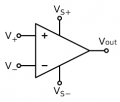Here the G of the + input to output is same as negative to output.
So Vout = G x ( V+ - V-). If V+ and V- are both the same then Vout
= 0 . The amp amplifies the difference in V its fed. But we have the CM
V as well to deal with.

Now in our case

VnodeA = V1 + V2
VnodeB = V1 + V3

If we connect these nodes to diff amp then

Vout = G x [VnodeA - VnodeB] = G [(V1 + V2) - (V1 + V3)] = G x (V2 - V3), we have
rejected in output the common mode V1. In this case we have the difference V
of the two T sensors, which we wanted.

CM voltages or currents can be due to ohmic connection, induced EMI into conductors,
light affecting sensors, all kinds of things. So we are "usually" trying to get rid of it in our
measuring systems. But once in a while clever design makes use of CM voltages, and we
do not want to reject it. In out example we were only interested in the T sensor Voltages,
and we connected them with 2 wires each sharing one side as common. I saw a clever
design, in an LVDS backplane, where the CM signal was used for a system control, so it
was extracted thru magnetics, while still delivering the LVDS differential Voltages connected

Point of this is it does not take a diff amp to manage CM signals, one can use magnetics as
well. I am sure there are other techniques used as well.

One other thing. Amps that we use do not have perfect G matching + and - signal paths.
So there is a spec, CMR (Common Mode Rejection) and CMRR (Common Mode Rejection
Ratio) that show us they are imperfect and have limitations to rejecting CM signals. When
using magnetics the CMR and CMRR would be impacted by windings that are not precisely
symmetrical, for example. There is even a spec on the PSRR (Power Supply Rejection Ratio)
which impacts noise fed to output of amp from its power supply rails.

AC power lines can have CM problems due to unbalanced loads, so that one phase is not same
as another phase in V.

Lastly CMR and CMRR in amplifiers is frequency sensitive, so what looks good at low freq starts
evaporating on designer as signals go up in freq. Usually there are curves of this published in
datasheet. Run of the mill opamps at low freqs have 60 - 70 db of CMRR, real high performance
stuff (usually laser trimmed on production line) can have 120 - 130 db, pretty awesome. Like if you
have a 1 V CM signal, and its 120 db down due to CMR, its error is only 1 uV in output amp error.

Regards, Dana.

Last edited:
•MikeJacobs and atferrari

#### OBW0549

Joined Mar 2, 2015
3,545
So how do these two things connect. How does this video about common mode voltage apply to parts and this example I gave on the comparator spec?
I think the problem here is that the phrase "common mode voltage" has two (at least) somewhat different meanings depending on context.

I only watched brief snippets of the video, but he appears to be talking about CMV with respect to amplifying or detecting signals; a signal of interest may appear as a differential signal (that is, a voltage difference between the two inputs), and we want to process that signal while rejecting common-mode signals (the voltage of the two inputs relative to circuit common ("ground"). In op amps, that rejection is embodied in the data sheet spec called "common mode rejection ratio."

But "common mode voltage" has another, entirely different, meaning which is the one I think you're referring to. For any op amp or comparator there is a range of input voltages, relative to the supply terminals, over which the part will function correctly; this is called the "input common mode voltage range" or simply "input voltage range" on the data sheet. To work correctly, an op amp's (or comparator's) inputs must ALWAYS be made to fall within that range-- under all conditions and at all times. If they don't, the part either will not function or, in the worst case, it may vent its Magic Smoke and stop working altogether.

For old-fashioned op amps such as the 741, that input voltage range went from a couple of volts above the negative supply voltage to a couple of volts below the positive supply voltage. This is one reason why 741-type op amps don't work in circuits with a single 5 volt supply; there's no room for the signals to swing.

For so-called "single supply" op amps, such as the 324 or 358, the input voltage range goes from the negative supply voltage (usually ground) up to within a volt or two of the positive supply voltage. This makes them easier to use in single-supply applications.

And for so-called "rail-to-rail input" op amps, the input voltage range spans the full supply voltage range (or even slightly beyond). As long as the inputs are kept "within the rails" the part will work correctly.

Hope this clears up the issue a bit...

•MikeJacobs and hrs

Joined Mar 10, 2018
4,057
Comparator detection is multifacted.

First you have the overdrive issue. If G of comparator is, for example, 100,000, and you want output
to go from 0 to 5 V, then min signal is 5V / 1E5 = 50 uV. But then you have offsets to consider, and
noise. Then if comparator is not overdriven, much more V that whats needed, it takes a long time for
comparator to achieve final output, non withstanding even start oscillating on you.

https://www.analog.com/media/en/training-seminars/tutorials/MT-083.pdf

https://www.maximintegrated.com/en/design/technical-documents/tutorials/8/886.html

Regards, Dana.

#### crutschow

Joined Mar 14, 2008
25,392
Basically for a two wire signal, common-mode is the voltage that is common (the same from both wires to ground).
This is normally desired to be suppressed as compared to the signal voltage.
In amplifiers this is called common-mode rejection.

Differential-mode is the voltage difference between them (normally the signal voltage).
Amplifiers normally amplify this voltage.

#### atferrari

Joined Jan 6, 2004
4,090
I do not intend to derail this thread at all. If required by @MikeJacobs I will start a new one.

I always found hard to grasp the concept of CM and DM. Yesterday I was about to post basically similar questions.

As per the video, CM is a conceptual value and not something I could measure but just calculate. It explicitly says it has nothing to do with offset of the input signal (!!??) At lost again.

In the attached file, I implemented the explanation by danadak and NodeC gives 5V. But given my fixation with the long tail pair used in opamps as the front end, I went with the skinny circuit on the right showing the equivalent NodeCLT with 0V.

@danadak, could you show, in the same .asc if you prefer (please delete whatever is wrong), two complete example with values, showing CM and DM for varying DC and AC input signals?

#### Attachments

• 1.8 KB Views: 5
Last edited:

#### crutschow

Joined Mar 14, 2008
25,392
It explicitly says it has nothing to do with offset of the input signal
Yes, offset would be a differential signal, not common-mode.

Joined Mar 10, 2018
4,057
1 V of common mode (V3), no differential mode V (V4), output tiny due to offset/noise/finite OpAmp internal G.

The OpAmp in differential mode has essentially rejected the common mode input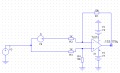1 V of common mode, 1 V AC differential, output 10V due to circuit G of 10, due to R1 - R4.

The OpAmp in differential mode still rejected the common mode but amped the AC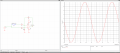If I offset the AC in, by 1V, then the output would be offset by 10V because the offset in this case looks like any diff signal. Its not CM to
both inputs any longer. The inputs see this as a differential signal, both AC and DC.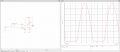The output now shifted down because of V4 offset and sign, but output now clipping because its running out of power supply headroom.

So in short a diff mode amplifier rejects signals AC or DC that are common mode, and amps signals that are diff mode, AC or DC.
Limited by power supply rails, and frequency for AC because OpAmp runs out of G at high freq and CM performance drops due
to lower G internal.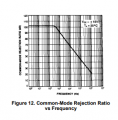Regards, Dana.

#### Attachments

Last edited:
•atferrari

#### MikeJacobs

Joined Dec 7, 2019
162
Ok so before I get totally confused
First of all, thanks to everyones inputs. Such an amazing response here.

Let me ask you this example. Maybe it will help me
Say you are measuring current. with a shunt resistor and a diff amp on he high side of some power circuit.

The diff amp is just looking at the diff voltage. No problem that makes perfect sense.

But what is the common mode voltage here? Say this is just a DC signal.

its 10V supply and a 50 mO shunt resistor in series and we have a diff amp across it.

What is the common mode and what is the diff mode voltages in this case?

there is only one signal V1 that is 10V

There is no v2???? So how does it work in this context?

#### crutschow

Joined Mar 14, 2008
25,392
What is the common mode and what is the diff mode voltages in this case?
If you are referencing the voltage measurements to ground (which is the usual way), then the common-mode voltage is 10V.
So the differential amp has to operate properly with that common-mode voltage.

The differential voltage is the signal voltage across the shunt resistor due to the current.

#### atferrari

Joined Jan 6, 2004
4,090

All clear now. I appreciate the time and effort you spend in replying.

#### kubeek

Joined Sep 20, 2005
5,732
Lets try to make it simple. In your case of a high-side shunt on a 10V power supply, lets assume the negative supply pin of the measuring opamp is connected to negative side of the of that power supply.

That negative supply point is common to both the power supply and the opamp, and that is what is meant by the common voltage range. In this differential amp arrangement using any opamp, but in the latest picture TL072, there is a limit to where the positive input and negative input can be realative to the supply pins of that opamp, so it still produces a valid resutlt. If i remember correctly TL072 need the both inputs to be roughly 2V higher than the negative supply , and also 2V lower than the positive supply in order to function properly

If you achive this by design, then you have to deal with what the output of the opamp can do. For an opamp like this, it can also only reach about 1-2V below the upper supply and 1-2V above the lower supply

#### WBahn

Joined Mar 31, 2012
26,143

I don't know if the equations will render for you correctly or not. Some browsers changed their MimeTex rendering engine a few years back and so it's hit an miss. Chrome (at least my version) doesn't.

But the basic idea is pretty simple.

If I have two signals, v1(t) and v2(t), then it is not uncommon for me to be primarily interested in the difference of the two signals

vd = v2(t) - v1(t)

I would like to represent v1(t) and v2(t) in terms of vd(t). I therefore attribute half of the difference to each signal and write

v2(t) = +vd(t)/2 + something1
v1(t) = - vd(t)/2 + something2

A bit of algebra should convince you that

something1 = something2 = (v2(t) + v1(t))/2 = the average of v1(t) and v2(t).

We call the average signal the "common mode" signal.

At the end of the day, all we are saying is that we can represent two arbitrary signals in terms of the average (what they have in common) plus/minus half of the difference (what they don't have in common).

But, as OBW0549 said, data sheets are often a bit sloppy and talk about the "input common mode range" when that is not what they really mean. Instead, what they almost always mean is the input voltage range of each signal, completely irrespective of any other signal, relative to that chip's power supply rails.

•atferrari

#### MisterBill2

Joined Jan 23, 2018
6,997
Basically for a two wire signal, common-mode is the voltage that is common (the same from both wires to ground).
This is normally desired to be suppressed as compared to the signal voltage.
In amplifiers this is called common-mode rejection.

Differential-mode is the voltage difference between them (normally the signal voltage).
Amplifiers normally amplify this voltage.
Both post #3 and post #5 are correct. Post #5 is a lot simpler.
Differential voltage is the difference between the two conductors that are not the common reference, which is usually "ground", in any of the meanings. Then common mode voltage is the voltage between tat pair of wires and that ground. Where problems happen is when the voltage of interest is from the circuit between the two wires, and it is small, while the voltage between those two wires and ground is larger, especially when it is more than the device the wires are connected to can handle. Hopefully this added explanation helps. Note that I never mentioned amplifiers. They are not the only things that get connected to differential pairs. Telephones were the first type of devices connected to differential pairs.

#### crutschow

Joined Mar 14, 2008
25,392
They are not the only things that get connected to differential pairs. Telephones were the first type of devices connected to differential pairs.
And a transformer is another example of a device that rejects common-mode voltage while transmitting the differential-mode voltage.

•atferrari